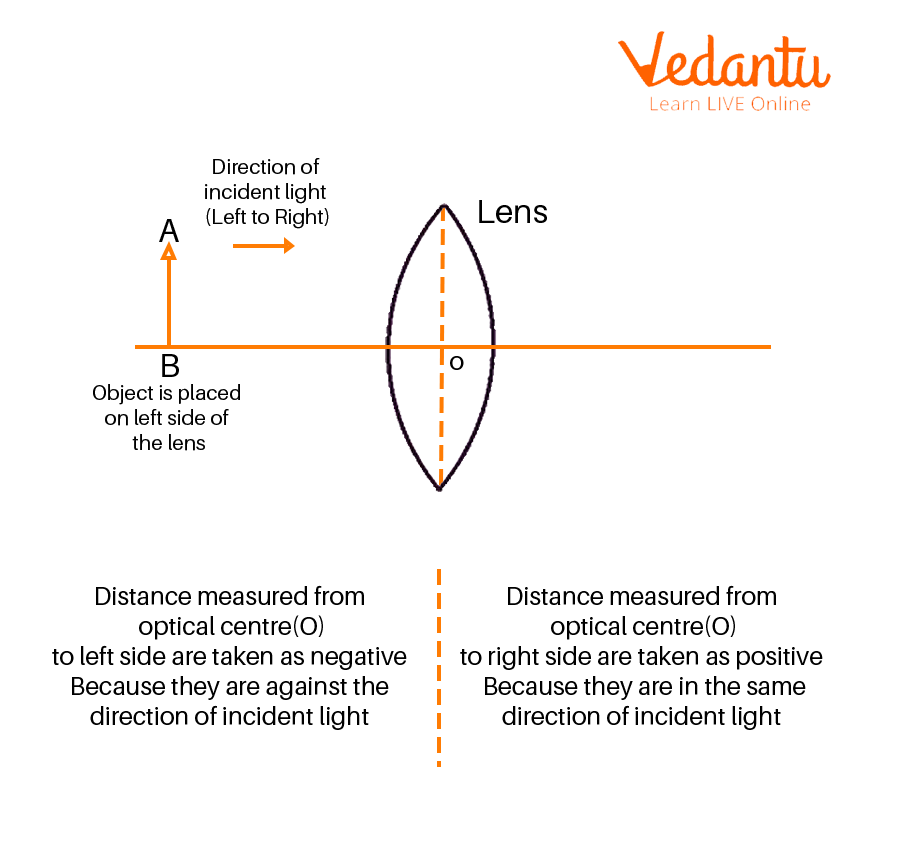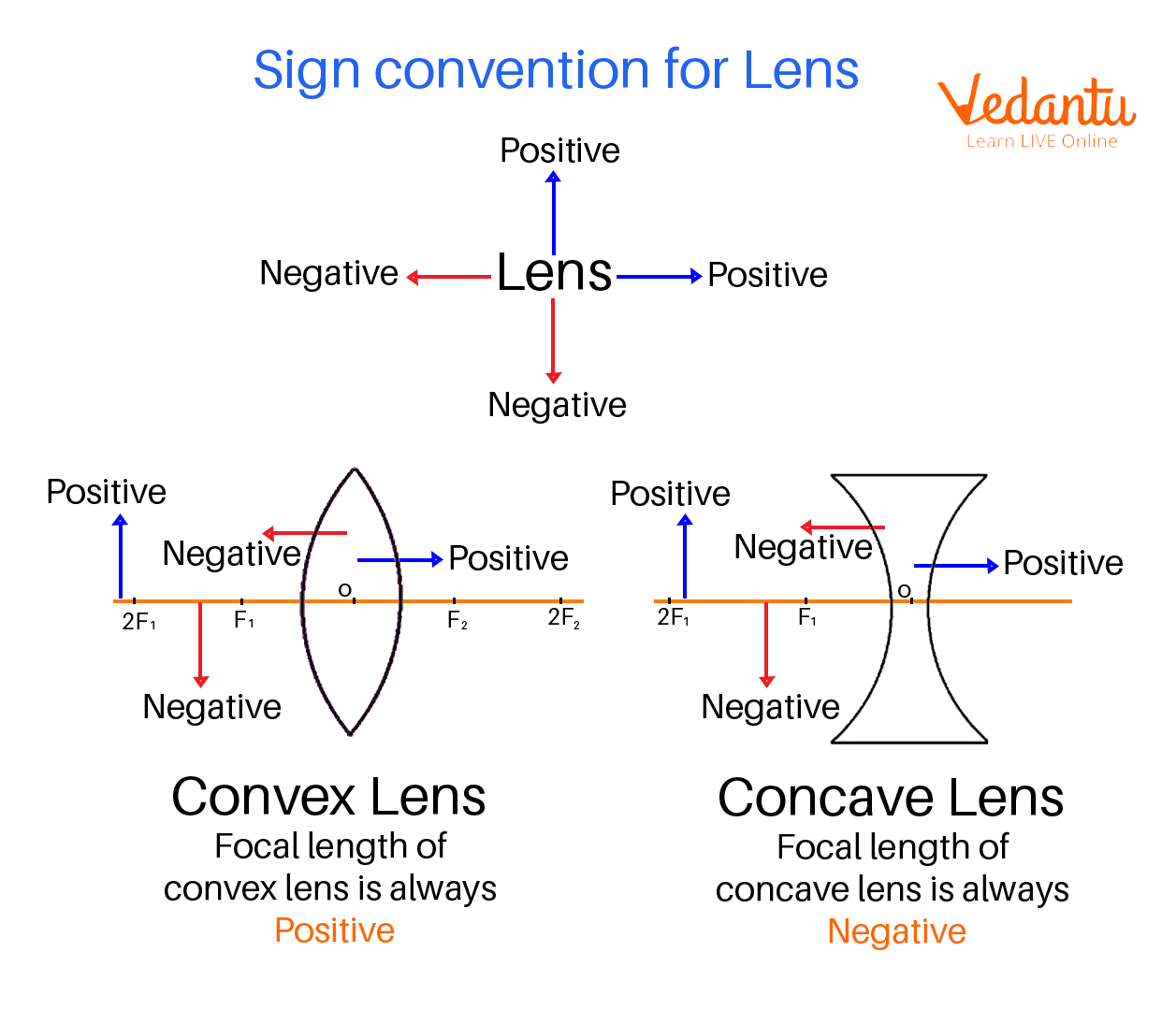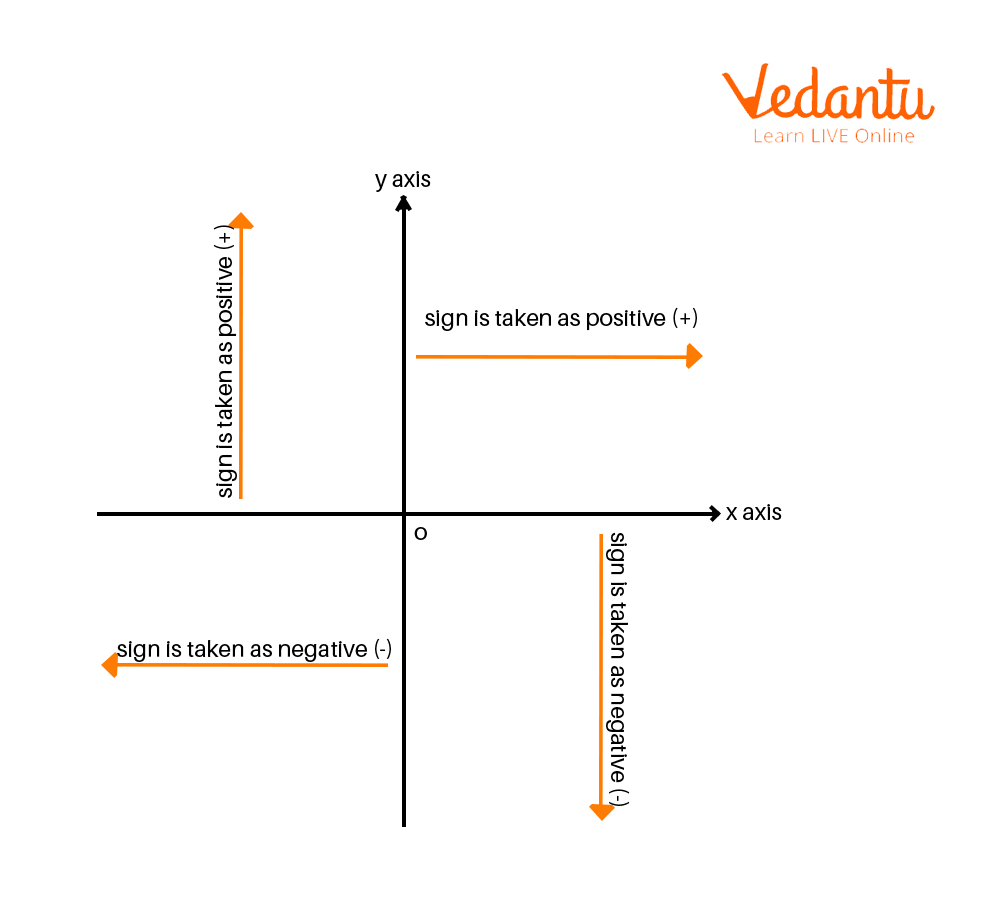Courses
Courses for Kids
Free study material
Free LIVE classes
More

# Sign Convention for Spherical LensLIVE
Join Vedantu’s FREE Mastercalss

## An Introduction to Spherical Lens

In physics, a sign convention is a choice of the physical significance of signs (plus or minus) for a set of quantities, in a case where the choice of sign is arbitrary. "Arbitrary" here means that the same physical system can be correctly described using different choices for the signs, as long as one set of definitions is used consistently. The choices made may differ between authors. Disagreement about sign conventions is a frequent source of confusion, misunderstandings, and even outright errors in scientific work.

In general, a sign convention is a special case of a choice of coordinate system for the case of one dimension. Sometimes, the term "sign convention" is used more broadly to include factors of i and 2π, rather than just choices of sign.The Spherical Lens

## Sign Convention for Concave and Convex Lens

 Convex Lens Concave Lens A convex lens is made up of two spherical surfaces that are curved outwards. This lens converges all paralle beams that approach it, passes through the lens, and focuses them all at the convex lens's focus.Focal length of the convex lens is positive. A concave lens is one that is made up of two spherical surfaces that are curved inwards. This lens diverges all parallel beams that approach it, pass through it, and then diverge again.Focal length of concave lens is negative.Sign Convention for Lens

The picture of the convex and concave lens shows that the lens has an optical centre at O, two foci F1 and F2, and two centres of curvature of the lens 2F1 and 2F2. The primary axis is a line that runs through the centre of curvature, foci, and optical centre. The optical centre is the place where the primary axis meets the lens. Some more important points to note about Sign Convention of spherical lenses:

• Since the object is always in front of the lens, object distance is always negative.

• If a real image is formed, the image is formed on the right side, so image distance is positive. If a virtual image is formed, the image is formed on the left side, so the image distance is negative.

• Since the object is always above the principal axis, the object height will be positive. If the image is above the principal axis, the image height will be positive. It means that the image is erect . If the image is below the principal axis, image height will be negative. It means that image is inverted

## Sign Convention and Lens Formula

The same sign convention is used for lenses as it is for spherical mirrors. We use the same criteria for distance signs, except that all measurements are measured from the optical centre of the lens. According to tradition, the focal length of a convex lens is always positive, while that of a concave lens is always negative. You must use suitable signs for the values $u,\text{ }v,\text{ }f,$object height $h,$and image height $h'.$

## Sign ConventionThe Sign Conventions

Cartesian sign conventions are used to quantify the different distances in the ray diagrams of spherical lenses.

• The object is always positioned on the lens's left side, according to the Cartesian sign convention.

• All distances are measured from the optical centre of the lens.

• Positive distances are measured in the direction of the incident light.

• Negative distances are those measured in the direction opposite the incident light.

• Positive distances are those that are measured upward and perpendicular to the primary axis.

• Distances measured downhill and perpendicular to the primary axis are awarded negative values.

## The Lens Formula

We have a formula for spherical lenses, just as we do for spherical mirrors. This formula gives the relationship between object distance$(u)$, image distance, $(v)$ and focal length$(f)$. The lens formula is expressed as:

$\frac{1}{f}=\frac{1}{v}-\frac{1}{u}$

The lens formula given above is general and is valid in all situations for any spherical lens. Take proper care of the signs of different quantities while putting numerical values for solving problems relating to lenses.

## Power of Lens and Magnification

The power of a lens is the measure of the degree of convergence or divergence of the light rays falling on it. The degree of convergence or divergence depends upon the focal length of the lens. Thus, we define the power of the lens as the reciprocal of the focal length of the lens used. It is given as

${\frac{{1}}{{f}}}$

The magnification of a lens is defined as the ratio of the height of an image to the height of an object. It is also given in terms of image distance and object distance. It is equal to the ratio of image distance to that of object distance.

$m = {\frac{{h}_{‘}}{{h}}} ={\frac{{u}}{{v}}}$

Where

• m= magnification

• h’= height of the image

• h = height of an object

• v is the image distance

• u is the object distance

## Convention Table for Lens Signs

 Quantity Condition Sign Focal Length, f Convex LensConcave Lens Positive (+)Negative (-) Object Distance Always Negative (-) Image Distance Image RealImage Virtual Positive (+)Negative (-) Magnification Image UprightImage Upturn Positive (+)Negative (-)

## Solved Examples

1. A plano-convex lens with a focal length of 30 cm has had its flat surface silvered. An object is placed 40 cm from the lens on the convex side. How far away is the image?

Ans: We know that, from lens formula:

${\frac{{1}}{{v}}}- {\frac{{1}}{{u}}} = {\frac{{1}}{{f}}}$

${\frac{{1}}{{v}}} = {\frac{{1}}{{30}}} - {\frac{{1}}{{40}}}$

${\frac{{1}}{{v}}} = 4 - {\frac{{3}}{{120}}}$

v = +120 cm

So now, this image will act as an object for the lens.

As a result, u = +120 cm and f = 30 cm

${\frac{{1}}{{v}}} - {\frac{{1}}{{u}}} = 1 - f$

${\frac{{1}}{{v}} {=} \frac{{1}}{{30}} {+} \frac{{1}}{{120}}}$ cm

v = +24 cm

2. A point object O is 30 cm away from a convex lens (focal length 20 cm) that has been split into two pieces, each displaced by 0.05 cm perpendicular to the main axis. What is the distance between the two images?

Ans: We will assume that each part as a separate lens with:

u = -30 cm and f = 20 cm.

Now, we know that,

${\frac{{1}}{{v}}}- {\frac{{1}}{{u}}} ={\frac{{1}}{{f}}}$

${\frac{{1}}{{v}}}- (-{\frac{{1}}{{30}}}) = -120$

v = 60 cm

Therefore, each part will form a real image of the point object O at 60 cm from the lens.

Now, from magnification formula we have:

$m = {\frac{{v}}{{u}}} = {\frac{{h}_{i}}{{h}_{o}}}$

${\frac{{60}}{{30}}} = {\frac{{h}_{i}}{{0.05}}}$

${{h}_{i}}$ = 0.1 cm

Thus, the first image will be 0.1 cm above the first half of the lens (say L1) optic axis and the second image will be 0.1 cm above the second half of the lens (say L2) optic axis. Also, separation between the two lenses = 0.05+0.05= 0.1 cm

We can say that

Distance between the two images = 0.1 + 0.1+0.1 =0.3 cm

## Summary

The sign convention in lenses is vital for picture quality and formation. This article has provided all of the pertinent information on the subject. The sign convention is required to determine the orientation of the picture. In addition, the article informs us about the working principles and properties of various lenses. The behaviour of light as it passes through the convex and concave lenses differs.

Convex lenses behave like converging lenses because they converge the light and form images, whereas concave lenses behave like diverging lenses because they diverge the incoming light and produce images. We also learnt about the lens sign convention table and had a firm grasp on the magnification sign convention.

Last updated date: 22nd Sep 2023
Total views: 92.7k
Views today: 1.92k

## FAQs on Sign Convention for Spherical Lens

1. Can a convex lens provide an image the same size as the object?

When an item is put at a distance twice its focal length, u=2f, a convex lens generates an image the same size as the thing. “u” is the object's distance from the lens's optical centre. The image created by the convex lens will be the same size as the item. The image creation is determined by the distance between the object and the focal length of the lens.

2. Write a few applications for a mirror and a lens.

The applications of a lens are listed below:

• Lenses are used in microscopes.

• Wearing glasses or spectacles.

• Telescopes are made up of lenses.

• Lens is used in magnifying glasses.

• Attenuate light

The applications of a mirror are listed below:

• Mirrors are used in automobile headlights.

• Solar appliances also use mirrors.

• Our houses have mirrors which we use daily.

• Different mirrors are used in photography.

• Energy and affirmation techniques.

• Decorations also use mirrors as they reflect light and create an illusion of open space by doubling whatever is present in the room.

3. Why and how does the lens bend light?

We know that every material is made of electrons. Now, when two matter interact, the electrons will also undergo the interaction and their behaviour is manifested as the phenomenon we get to observe. So, when the light passes closely around any material, the electrons inside the material get strongly affected and the influence gives the light rays a bent trajectory. The measure of how much a material can bend a light ray is expressed via its refractive index.